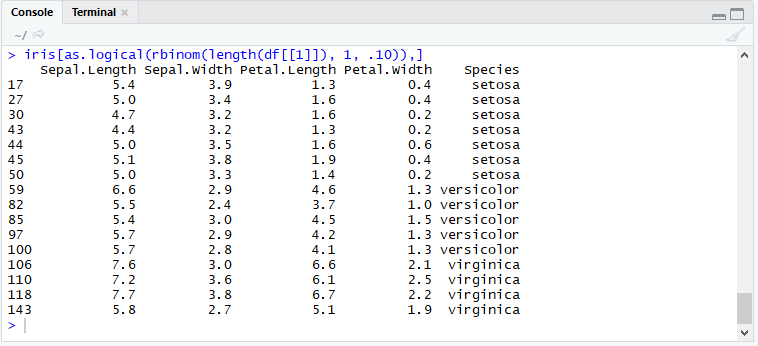}

# Randomly Sampling Rows in R

2019-05-22

It's impossible to imagine a data scientist who does not have to randomly sample datasets on a regular basis. Most employ the useful and easy function sample( ), defined in R's base namespace. Let's take a closer look at sample( ) and then take a look at a flexible alternative that is just as easy and quick to use.

The sample function takes a random sample of a vector, not a dataframe. This is why the most commonly used pattern looks like this:

iris.sampled<-iris[sample(1:nrow(iris),30, replace=FALSE),]

To fully appreciate what this line of R code is doing, let's break it down into three separate statements:

# create a vector the same length as the dataframe

the_vector<-1:nrow(iris)

# sample elements from the vector (in this example 30 elements sampled without replacement)

the_sample<- sample(the_vector,30, replace=FALSE)

# the vector of randomly selected elements is then used to select rows from the dataframe

iris.sampled<-iris[the_sample,]

We could, if the need arose, directly create a sample from a vector. This will only work with vectors, not with a dataframe.

Sepal.Length.sampled<-sample(iris[,"Sepal.Length"],30)

## A Direct "Hands-On" Approach

We don't actually need the sample( ) function at all. In fact, a direct approach can have the advantage of being more flexible if one should require a customized approach to sampling. Let's take a moment to review binomial( ), one of R's generators for random numbers.

The following example generates the numerical equivalent of tossing four pennies, recording the number of heads, and repeating the experiment 50 times.

`rbinom(50, 4, .5)`

If we are sampling rows, we only want the equivalent of one penny. Heads we take the row, tails we leave it behind.

rbinom(length(df[]), 1, .10)

In the above example, we are only planning to take one row in ten, as if the coin had only a 10% chance of coming up heads. rbinom( ) returns integers, however, and if we plug rbinom( ) into a dataframe we will get row one a whole bunch of times.

iris[rbinom(length(df[]), 1, .10),] # wrong

What we need is a logical vector, telling us whether an individual row should be selected, not an integer vector of row numbers.

iris[as.logical(rbinom(length(df[]), 1, .10)),]

Now we have the subset we want.[sidebar_cta header="Data Science is More Than a Buzzword. It's the Key to Your Organization's Long-Term Success." color="blue" icon="" btn_href="https://www.learningtree.com/resources-library/webinars/data-science-demystified-informed-organizational-decision-making/" btn_href_en="https://www.learningtree.com/resources-library/webinars/data-science-demystified-informed-organizational-decision-making/" btn_href_ca="https://www.learningtree.ca/resources-library/webinars/data-science-demystified-informed-organizational-decision-making/" btn_href_uk="https://www.learningtree.co.uk/resources-library/webinars/data-science-demystified-informed-organisational-decision-making/" btn_href_se="https://www.learningtree.se/kunskapsbank/webinars/data-science-demystified-informed-organisational-decision-making/" btn_text=" Learn More, Watch Our On-Demand Webinar"]

## Splitting a Dataframe into Training and Testing Sets

One of the most practical illustrations of the flexibility of this technique is the ease with which we can split a dataframe into training and testing sets without invoking an external package. Since we already have the logical vector, we can use the vector and its logical opposite to create the two sets we need.

random.logical_vector<-as.logical(rbinom(length(df[]), 1, .80))

training <- iris[random.logical_vector,]

testing <- iris[!random.logical_vector,]

Curiously, we could create a random logical vector using the sample function.

random.logical_vector <- sample(c(TRUE, FALSE), nrow(df), replace = T, prob = c(0.6,0.4))

Note that in this case, we sample from a vector with only two elements, TRUE and FALSE. Clearly, to obtain the random vector we need, we need to sample with replacement.

## Conclusion

Manually creating a random logical vector for the sampling of R dataframe rows is no more difficult than using the sample( ) function and can be far more flexible. Using a logical vector, we can easily split a dataframe into training and testing sets without loading any external libraries.### Written by Dan Buskirk

The pleasures of the table belong to all ages.” Actually, Brillat-Savaron was talking about the dinner table, but the quote applies equally well to Dan’s other big interest, tables of data. Dan has worked with Microsoft Excel since the Dark Ages and has utilized SQL Server since Windows NT first became available to developers as a beta (it was 32 bits! wow!). Since then, Dan has helped corporations and government agencies gather, store, and analyze data and has also taught and mentored their teams using the Microsoft Business Intelligence Stack to impose order on chaos. Dan has taught Learning Tree in Learning Tree’s SQL Server & Microsoft Office curriculums for over 14 years. In addition to his professional data and analysis work, Dan is a proponent of functional programming techniques in general, especially Microsoft’s new .NET functional language F#. Dan enjoys speaking at .NET and F# user’s groups on these topics.

Chat With Us